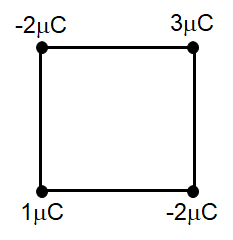Electric dipole
Question

# Four point charges $1\mu C,-2\mu C,3\mu C$  and $-2\mu C$  are arranged on the four vertices of a square of side 1 cm.  The dipole moment of this charge assembly isEasy
Solution

## $\stackrel{\to }{\mathrm{p}}=\sum {\mathrm{q}}_{\mathrm{i}}\stackrel{\to }{{\mathrm{r}}_{\mathrm{i}}}$ we can rearrange the charges to form three dipoles with charges as  (-2 and +2) ,(+1 and _1) and (-1 and +1) with first and third in opposite direction and second one is perpendicular to them.

Get Instant Solutions